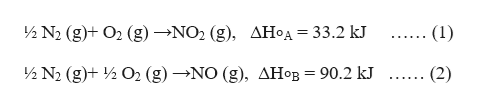Calculate the enthalpy of the reaction2NO(g)+O2(g)→2NO2(g)given the following reactions and enthalpies of formation:12N2(g)+O2(g)→NO2(g),   ΔH∘A=33.2 kJ12N2(g)+12O2(g)→NO(g),  ΔH∘B=90.2 kJCalculate the enthalpy of the reaction4B(s)+3O2(g)→2B2O3(s)given the following pertinent information:B2O3(s)+3H2O(g)→3O2(g)+B2H6(g),    ΔH∘A=+2035 kJ2B(s)+3H2(g)→B2H6(g),                             ΔH∘B=+36 kJH2(g)+12O2(g)→H2O(l),                             ΔH∘C=−285 kJH2O(l)→H2O(g),                                           ΔH∘D=+44 kJ

Question

Calculate the enthalpy of the reaction

2NO(g)+O2(g)→2NO2(g)

given the following reactions and enthalpies of formation:

1. 12N2(g)+O2(g)→NO2(g),   ΔH∘A=33.2 kJ
2. 12N2(g)+12O2(g)→NO(g),  ΔH∘B=90.2 kJ

Calculate the enthalpy of the reaction

4B(s)+3O2(g)→2B2O3(s)

given the following pertinent information:

1. B2O3(s)+3H2O(g)→3O2(g)+B2H6(g),    ΔH∘A=+2035 kJ
2. 2B(s)+3H2(g)→B2H6(g),                             ΔH∘B=+36 kJ
3. H2(g)+12O2(g)→H2O(l),                             ΔH∘C=−285 kJ
4. H2O(l)→H2O(g),                                           ΔH∘D=+44 kJ
Step 1

Hi there! Since there are multiple questions, we will answer the first question for you. If you need help with the other question, kindly post it separately.

Step 2

It is required to calculate the enthalpy of the reaction which is,

Step 3

The given reacti...help_outlineImage Transcriptionclose½ N2 (g)+ O2 (g)-NO2 (g), AHA = 33.2 kJ .. (1) ½ N2 (g)+2O2 (g)-»NO (g), AHOB = 90.2 kJ (2) fullscreen

Want to see the full answer?

See Solution

Want to see this answer and more?

Our solutions are written by experts, many with advanced degrees, and available 24/7

See Solution
Tagged in

Chemical Thermodynamics